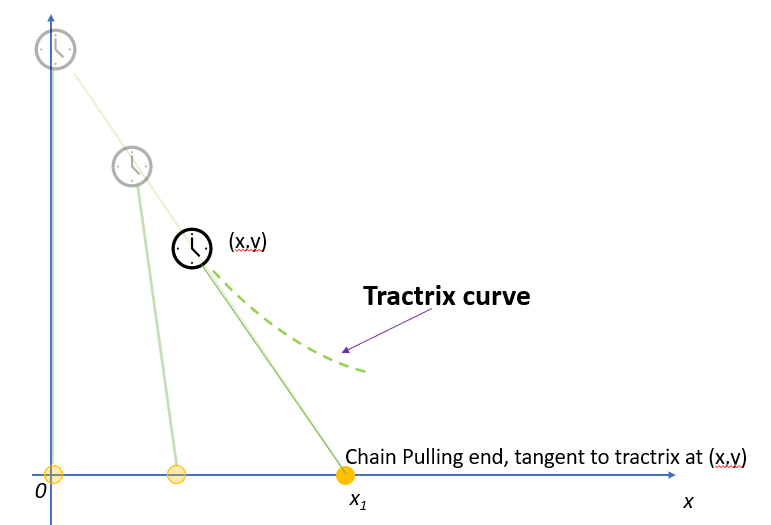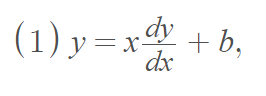# Tractrix Curve: Definition, Example, and Formula

A tractrix curve is the curve created by a moving object that is dragged along a horizontal plane by a taut string or chain.

One of the earliest demonstrations of the tractrix curve was in 1693 by French architect Claude Perrault. He laid his watch on the table with the chain in a straight line (back in those days, watches were attached to chains, not wrists!). He demonstrated that when he moved the end of the taut chain along a straight line, the watch was dragged along a curve — which we now call the tractrix .Although the terms tractrix curve and pursuit curve are often used synonymously, the tractrix is a type of pursuit curve. Pursuit curves can take on many different curves, while a tractrix curve is usually described by the specific example of the watch being pulled by a chain. The tractrix itself is the equation for the curve .

## Tractrix Curve: Finding the EquationLet xi define the location of the chain’s pulling end. As the chain end is traveling along the x-axis, y = 0, so:Next, define the equation of the tangent line that intersects the x-axis at xi:The Pythagorean theorem tells us that:Take the positive square root of both sides (note that the tractrix slope is negative):Separate the variables:Find the antiderivative:Since y(x = 0) = a (where a is the length of the chain), we have C = 0 (C is the constant of integration). Rewriting, we get the equation for the tractrix curve:As an example, the graph of the tractrix curve (graphed with Desmos.com) with a = 2 is:## References

 W. G. Cady. The circular tractrix. Amer. Math. Monthly, 72:1065–1071, 1965.
 Moiseeva, S. (2011). Optimal control problems, curves of pursuit. University of New Mexico. UNM Digital Repository.

CITE THIS AS:
Stephanie Glen. "Tractrix Curve: Definition, Example, and Formula" From StatisticsHowTo.com: Elementary Statistics for the rest of us! https://www.statisticshowto.com/tractrix-curve/